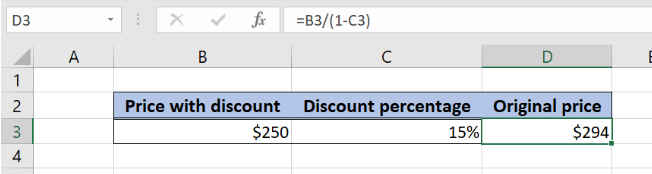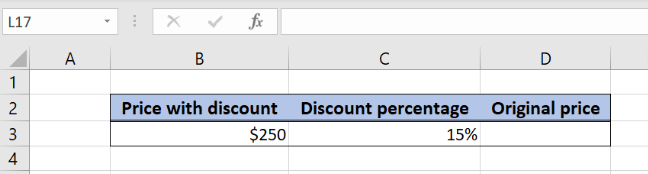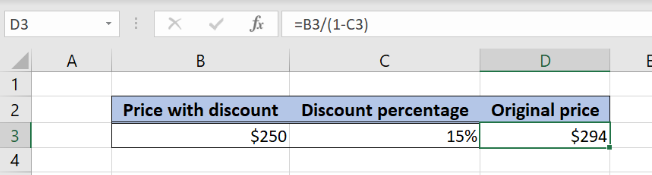Get instant live expert help with Excel or Google Sheets“My Excelchat expert helped me in less than 20 minutes, saving me what would have been 5 hours of work!”

#### Post your problem and you’ll get expert help in seconds.

Your message must be at least 40 characters
Our professional experts are available now. Your privacy is guaranteed.

# Get the Original Price from a Percentage Discount in Excel

Excel allows a user to calculate an original price from percentage discount using a simple formula. This step by step tutorial will assist all levels of Excel users in calculating an original price based on a price with a discount.Figure 1. The result of the formula

## Syntax of the Formula

The generic formula is:

`=price_with_discount / (1 - discount)`

The parameters of the formula are:

• price_with_discount – a price with discount for which we want to calculate the original price
• discount – a discount on a price in percentage

## Setting up Our Data for Calculating the Original PriceFigure 2. Data that we will use in the example

Let’s look at the structure of the data we will use. In the cell B2, we have the price with discount. In the cell C2, we have the discount in percentage. We want to get the original price in the cell D3.

## Calculating the Original Price without Discount

In our example, the price with discount is \$250, while the discount is 15%. Based on these two values, we want to calculate the original price, without the discount.Figure 3. Calculating the original price without discount

The formula looks like:

`=B3/(1-C3)`

The parameter price_with_discount is B3, while the parameter discount is C3.

Finally, the result in the cell D3 is -\$294, which is the original price, before applying the discount of 15%.

To apply the formula, we need to follow these steps:

• Select cell D3 and click on it
• Insert the formula: `=B3/(1-C3)`
• Press enter

Most of the time, the problem you will need to solve will be more complex than a simple application of a formula or function. If you want to save hours of research and frustration, try our live Excelchat service! Our Excel Experts are available 24/7 to answer any Excel question you may have. We guarantee a connection within 30 seconds and a customized solution within 20 minutes.

### Did this post not answer your question? Get a solution from connecting with the expert.Another blog reader asked this question today on Excelchat:## Subscribe to Excelchat.coAnother blog reader asked this question today on Excelchat: